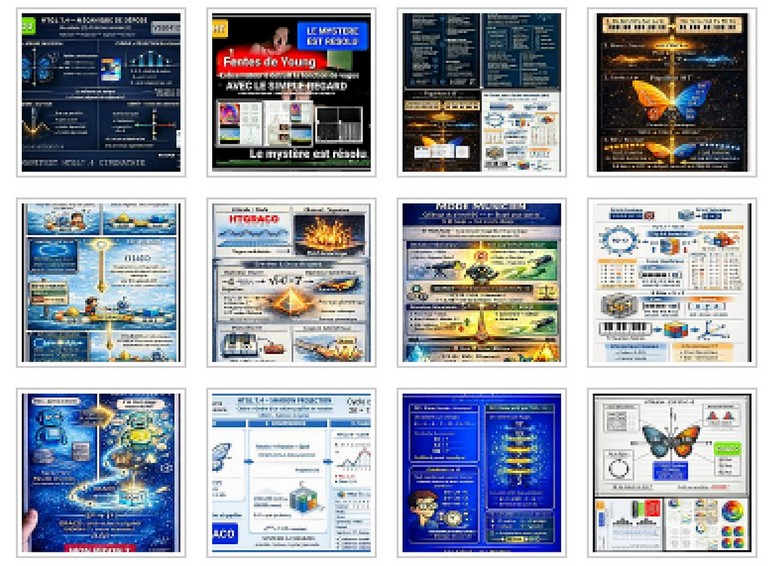## LICENCE D'EXPLOITATIONAfin d'obtenir une licence d'exploitation de l'université des sciences quantiques dans votre pays ou votre région, veuillez-nous contacter. Nous allons examiner votre demande et vous transmettre les informations nécessaires pour obtenir un licence d'exploitation UNIQ RBO_ISSOa.

## Unification theoryby an undulatory movement

HT introduces a constant in mathematics

From a continuous uniform motion to a quantified dynamic movement
F= m*a + m*AkPi (| Q1Q2|)

By using the constant [], HT allows to introduce a new dimension in mathematics, where the real numbers can be considered as a projection of their values in two dimensions. This projection makes it possible to visualize the waves, energies and forces that make up the universe. HT also offers a new interpretation of quantum physics, which provides a better understanding of quantum phenomena such as uncertainty, superposition, entanglement and non-locality. The wave approach to quantum physics proposed by HT makes it possible to visualize these phenomena in termsof wave motions, fields and forces. In sum, HT offers a powerful conceptual framework for explaining how the universe works at both quantum and macroscopic scales. This unified theory makes it possible to link the different fields of physics, cosmology and philosophy by proposing a coherent vision of our world.

The universe is an island of space-time (Higgs field) on an ocean of probabilities (information field)

C(UNIQ) - University;of Quantum Sciences
University of Quantum Sciences
-
HT introduces into mathematics a constant ❤ , an imaginary complex iT3=0 to slide the analysis towards wave geometry or also called quantum geometry. Just like Euler, a Swiss mathematician born in Basel in 1707, slid algebra into analysis. HT, through an operation of amplification and not an operation of power, HT, makes the analysis slide towards wave geometry or also called quantum geomety of Aladin. In physics, HT introduces a K IMAGINARY complex particle, the Kazzon (// CERN,_Ms should be 13 &! 12), a particle which interacts with the Higgs field AND the information field through a force (quantified A) symbolized by a , interaction force observed in the laboratory in 1986 by Dr. René Peoc'h at the Physics & Quantum Institute of Paris to give a new vision of the universe